Courses

# Test: Gravitation- 2

## 30 Questions MCQ Test Physics Class 11 | Test: Gravitation- 2

Description
This mock test of Test: Gravitation- 2 for Class 11 helps you for every Class 11 entrance exam. This contains 30 Multiple Choice Questions for Class 11 Test: Gravitation- 2 (mcq) to study with solutions a complete question bank. The solved questions answers in this Test: Gravitation- 2 quiz give you a good mix of easy questions and tough questions. Class 11 students definitely take this Test: Gravitation- 2 exercise for a better result in the exam. You can find other Test: Gravitation- 2 extra questions, long questions & short questions for Class 11 on EduRev as well by searching above.
QUESTION: 1

### To complete one revolution around Earth, communication satellites take

Solution:

A communications satellite is in a GEO (geostationary equatorial orbit) with a period of 24 hours. The spin rate ωs about its axis of symmetry is 1 revolution per minute, and the moment of inertia about the spin axis is 550 kg · m2.

QUESTION: 2

Solution:
QUESTION: 3

### The decrease in the value of g on going to a height R/2 above the earth's  surface will be

Solution:

g = GM​/R2
g at height h
gh​ = GM/(R+H)2
h = R/2
gh ​= GM/(R + R/2​)2 ​= GM/((9/4) (R2)) ​= 4/9g
Decrease in the value of g
g − 4/9g = 5/9 g

QUESTION: 4

At what altitude will the acceleration due to gravity be 25% of that at the earth's surface (given radius of earth is R) ?

Solution:

Force on the body placed on Earth's surface is
F = GMm/R2
But, F=mg hence,
mg = GMm​/R2
where, variables have their usual meanings.
gR= GM
Now, force on the body at geo-potential height say h (altitude) where the acceleration due to gravity is 25% of that at the earth's surface i.e.
25//100g ​= g/4​
Hence, we can write
g/4​ = GM/(R+h)2

g/4​ = gR2/(R+h)2

(R+h)= 4R2

Taking roots for both sides we get
R+h = 2R
h = R

QUESTION: 5

If the rotational motion of earth increases, then the weight of the body -

Solution: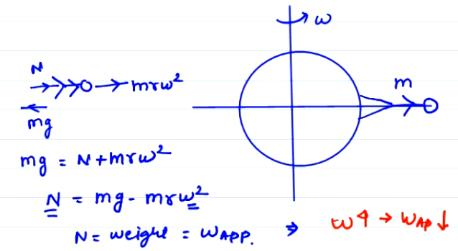QUESTION: 6

On doubling the distance between two masses the gravitational force between them will -

Solution:
QUESTION: 7

If the acceleration due to gravity inside the earth is to be kept constant, then the relation between the density d and the distance r from the centre of earth will be -

Solution:

If M is the mass of the Earth and m is the mass of a sphere of radius r and you want the force of gravity to be the same at both the surface of the sphere and the surface of the earth then mg = Mg and m = M. This means that the mass of the sphere would have to be the same as the mass of the Earth no matter what r is. This is impossible since a sub-sphere cannot have the same mass as its parent.
For a sphere of radius r to have force g at its surface, d = (De *Re)/r where De is the density of the entire Earth and Re is the radius of the Earth, d is the density of the sphere of radius r. So there is a radius and a density which will produce a gravitational force of g for any d, r >0 but one cannot be a subset of another.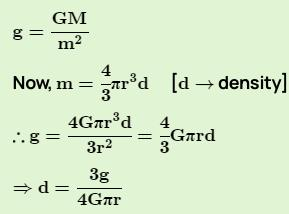= d μ 1/r

QUESTION: 8

Let w be the angular velocity of the earth's rotation about its axis. Assume that the acceleration due to gravity on the earth's surface has the same value at the equator and the poles. An object weighed at the equator gives the same reading as a reading taken at a depth d below earth's surface at a pole (d << R). The value of d is

Solution:

Let,  ge​ be the acceleration due to gravity on surface of earth, g′ be  the acceleration due to gravity at depth d below the surface of earth and is given by
g′=ge​(1−d/R​) ............................(1)
The acceleration due to gravity of an object on latitude(below the surface of earth) is
g′=ge​−ω2Rcosϕ
where, ϕ is the solid angle at the centre of earth sustained by the object.
At the equator, ϕ=0∘
∴cosϕ=1
Hence,
g′=ge​−ω2R ...............(2)
From (1) and (2), we can write
ge​(1−d/R​)=ge​−ω2
⇒dge​​=ω2R
⇒d= ​ω2R2​/ ge

QUESTION: 9

If the radius of the earth be increased by a factor of 5, by what factor its density be changed to keep the value of g the same ?

Solution:

The acceleration due to gravity on surface of earth is
g=GM​/ R2

g=GρV​/R2
where, G is the gravitational constant, M  is mass of earth, R  is radius of earth, ρ is the density and V is the volume of earth.
∴g=Gρ(4/3)​πR3​/ R2

∴g=(4/3)​GρπR
⇒g∝ρR
since, all other quantities are constant.
Hence, if the radius of the earth be increased by a factor of 5, density will decrease by 51​  to keep the value of g the same.

QUESTION: 10

The mass and diameter of a planet are twice those of earth. What will be the period of oscillation of a pendulum on this planet if it is a seconds pendulum on earth ?

Solution:

As Mp​=2Me​ and Dp​=2De
or Rp​=2Re​
Hence, gp​= GMp/(Rp​)2 ​​=G(2Me​)/(2Re​)2 ​= GMe/2Re2​ ​​=ge/2​​
Time period of pendulum on the planet  Tp​=2π√ l/gp​
Tp​=2π√​2l/ge​​=√2​×2π√l/ge​=√2​×Te
Tp​=√2​×2=2√2​s

QUESTION: 11

A particle of mass M is at a distance a from surface of a thin spherical shell of equal mass and having radius a.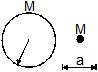Solution:

According to the question, there can be two cases in which the point mass is at a distance a from the surface of the spherical shell.

1. The point mass is at the center of the shell

2. The point mass is outside the shell at a distance 2a from  the center of the shell.

Superposition principle dictates that the potential and field at any point will be the sum of the potentials and fields respectively at that point due to the individual masses.

It can be seen that in both the cases, neither field nor potential is zero inside the shell. This is due to the contribution of the point mass.

QUESTION: 12

If the kinetic energy of a satellite orbiting around the earth is doubled then -

Solution:
QUESTION: 13

The escape velocity from a planet is v0. The escape velocity from a planet having twice the radius but same density will be -

Solution:
QUESTION: 14

Two planets A and B have the same material density. If the radius of A is twice that of B, then the ratio of the escape velocity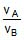is

Solution:

Let the density be d for both the planets. Given that RA​=2RB
Now, mass of A, MA​=4dπRA3/3​=32dπRB3​/3
similarly, MB​=4dπRB3/3
Escape velocity for a planet is given by V=√2GM​​/R
So, VA​=​√2GMA/3RA​​​=√​64GdπRB3/6RB ​​=√32GdπRB​2/3​​

Similarly, VB​=8GdπRB​2/3​​

Taking the ratio, ​VA/VB ​​=32GdπRB​2​/3​×​√3/8GdπRB​2​​=2

QUESTION: 15

A hollow spherical shell is compressed to half its radius. The gravitational potential at the centre

Solution:

Gravitational Potential V = -GM/R for hollow spherical shell at the centre. If we replace R by R/2 then we get V = -2GM/R. Therefore it decreases.

QUESTION: 16

A (nonrotating) star collapses onto itself from an initial radius Ri with its mass remaining unchanged. Which curve in figure best gives the gravitational acceleration ag on the surface of the star as a function of the radius of the star during the collapse ?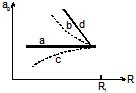Solution:

g∝1​/R2
Curve b represents this variation.

QUESTION: 17

A satellite of the earth is revolving in circular orbit with a uniform velocity V. If the gravitational force suddenly disappears, the statellite will

Solution:

Here the satellite is moving with constant velocity v and it is the gravitational force which is the necessary centripetal force to allow it to move around the earth.
Imagine this as: you are holding a string attached to a stone at other end and you whorl it in the air, now when you suddenly leave the string the stone will move outwards. This is actually tangential to the direction of previously applied force.
When this same concept is applied to the satellite, it will also move away tangentially and with uniform velocity due to zero net force in outer space and no air resistance (as space has vacuum).

QUESTION: 18

A satellite revolves in the geostationary orbit but in a direction east to west. The time interval between its successive passing about a point on the equator is

Solution: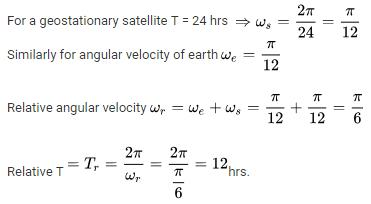QUESTION: 19

Two point masses of mass 4m and m respectively separated by d distance are revolving under mutual force of attraction. Ratio of their kinetic energies will be

Solution:

Let the velocity of m is v and velocity of 4m is u
Therefore, v = 4u and we know that kinetic energy is 1/2mv2 so for m:
KE = ½ x m x v2 = 1
And for 4m:
KE = ½ x 4m x 42 = 4
So, ratio of their kinetic energy is 1:4
Hence A

QUESTION: 20

Select the correct choice(s) :

Solution:

If the TE = 0 that means KE + PE = 0
KE = - PE which is enough for the body to leave the system (here, Earth)

QUESTION: 21

with the orbit radius of a body in circular planetary motion. Find the correct state ment about the

curves A, B and C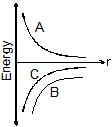Solution:

The body is in circular planetary motion this implies that Potential energy will be negative (attractive)  and the total energy of the system will lie between zero and potential energy (because of circular motion).

QUESTION: 22

Two different masses are dropped from same heights, then just before these strike the ground, the following is same :

Solution:

Both the objects with different masses will attain the same v when striking the ground because acceleration and height is the same.
As, K.E=(1​/2)mv2P.E=−GMm​p/r=mv
Thus K.E, P.E, and p will be different.

QUESTION: 23

When a satellite moves around the earth in a certain orbit, the quantity which remains constant is

Solution: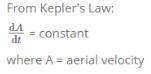QUESTION: 24

A body of mass m rises to height h = R/5 from the earth's surface, where R is earth's radius. If g is acceleration due to gravity at earth's surface, the increase in potential energy is

Solution:

Initial PE = -GMm/R
Final PE = -GMm/R+h, (h=R/5)
Increase in PE = final - initial
= -GMm/R + (R/5) - (-GMm/R)
=GMm(1/R - 5/6R)
=GMm/R * 1/6
= gmR/6 (g= GM/R)
=gm5h/6 (h=R/5)

QUESTION: 25

A planet has mass 1/10 of that of earth, while radius is 1/3 that of earth. If a person can throw a stone on earth surface to a height of 90m, then he will be able to throw the stone on that planet to a height

Solution:

Let mass of earth be M
Acceleration due to gravity = g
Radius of earth = r
Height to which he can throw on earth = H
Acceleration due to gravity at the planet be = g'
Radius of the planet be= r'
Mass of the planet be = M'
Height to which he can throw at that planet = H'
Then g = G(M/r^2)
Therefore g' = G{(1/10)M}/[{(1/3)r}^2]
= (9/10) G(M/r^2)
= (9/10)g
Now, H = {(v)^2-(u)^2}/2(-g)
= {(0)^2-(u)^2}/(-2g)
= {(u)^2}/2g
H' = u^2/[2{(9/10)g}]
= (10/9)u^2/2g
= (10/9)H
= (10/9) * 90
= 100 m

QUESTION: 26

Work done in taking a body of mass m to a height nR above surface of earth will be :

(R = radius of earth)

Solution:

Potential energy at surface of earth will be PE1 = (G.M.m)/R

Now, potential energy at height nR from the surface of earth,

PE2 = (G.M.m)/(R+nR)

Here, change in PE = PE1 – PE2

= (G.M.m)/R – (G.M.m)/(n+1)R

= (G.M.m)/R[1 – 1/(n+1)]

= (G.M.m)/R[(n + 1 – 1)/(n+1)]

= [n/(n+1)](G.M.m)/R ….(i)

Also, g = GM/(R^2) ….(ii)

From (i) and (ii),

Change in PE = (n/(n+1))mgR

QUESTION: 27

The potential energy of a body of mass 3kg on the surface of a planet is 54 joule. The escape velocity will be -

Solution:
QUESTION: 28

A body of mass m is situated at a distance 4Re above the earth's surface, where Re is the radius of earth. How much minimum energy be given to the body so that it may escape -

Solution:
QUESTION: 29

A satellite of earth is moving in its orbit with a constant speed v. If the gravity of earth suddenly vanishes, then this satellite will -

Solution:

Obviously, take example that u have one thread in your hand with stone tied at one end and your moving it in vertical circular manner and if u suddenly leave the thread it will go in the direction tangentially to your arm here your hand force is equal to gravitation force but your hand plays centrifugal and gravitation plays centripetal.

QUESTION: 30

The ratio of distances of satellites A and B from the centre of the earth is 1.4 : 1, then the ratio of energies of satellites B and A will be –

Solution:

We know that,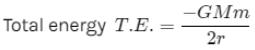Hence the ratio of total energy is in the inverse ratio of the distance ‘r’.